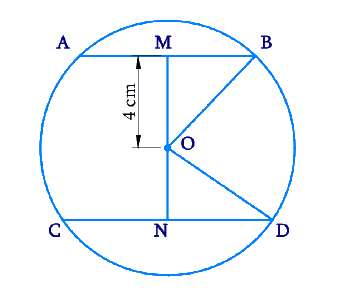# Ex.10.6 Q3 Circles Solution - NCERT Maths Class 9

Go back to  'Ex.10.6'

## Question

The lengths of two parallel chords of a circle are \begin{align} \text{6 cm}\end{align} and \begin{align} \text{8 cm.}\end{align} If the smaller chord is at distance \begin{align} \text{4 cm}\end{align} from the centre, what is the distance of the other chord from the centre?

Video Solution
Circles
Ex 10.6 | Question 3

## Text Solution

What is known?

Two parallel chords of lengths $$6\,\rm{ cm}$$ and $$8\,\rm{ cm}$$ and distance of smaller chord from centre is $$4\,\rm{cm.}$$

What is unknown?

Radius of the circle and distance of one chord from centre.

Reasoning:

The perpendicular drawn from the centre of the circle to the chords, bisects it.

Pythagoras theorem states that

(Side $$1)^{2}$$ $$+$$ (Side $$2)^{2} =$$ (Hypotenuse )$$^{2}$$

Steps:

Draw $$2$$ parallel chords \begin{align} {AB}\end{align} and \begin{align} {CD}\end{align} of lengths \begin{align} \text{6 cm}\end{align} and \begin{align} \text{8 cm.}\end{align} Let the centre of the circle be \begin{align} {O.}\end{align} Join one end of each chord to the centre. Draw $$2$$ perpendiculars \begin{align} {OM}\end{align} and \begin{align} {ON}\end{align} to \begin{align} {AB}\end{align} and \begin{align} {CD }\end{align} respectively which bisects the chords.\begin{align} {AB} &=6 \, \rm{cm} \\ {CD} &=8 \, \rm{cm} \\{MB} &=3 \, \rm{cm} \\ {ND} &=4 \,\rm{cm} \end{align}

Given $$OM = \text{4 cm }$$

and let $${ON =} x\, \rm{cm}$$

Consider \begin{align} \Delta {OMB}\end{align}

By Pythagoras theorem,

\begin{align}{OM}^{2}+{MB}^{2}&={OB}^{2} \\ 4^{2}+3^{2}&={OB}^{2} \\ 16+9&={OB}^{2} \\ {OB}^{2}&=25 \\ {OB}&=5 \,\mathrm{cm}\end{align}

\begin{align}{OB}\end{align} and \begin{align}{OD}\end{align} are the radii of the circle.

Therefore $$OD = OB =\text{ 5 cm.}$$

Consider \begin{align}\Delta{OND}\end{align}

By Pythagoras theorem,

\begin{align}{ON}^{2}+{ND}^{2}&={OD}^{2} \\ x^{2}+4^{2}&=5^{2} \\ x^{2}&=25-16 \\ x^{2}&=9 \\ x&=3 \, \mathrm{cm}\end{align}

The distance of the chord \begin{align}{CD}\end{align} from the centre is \begin{align}\text{3 cm.}\end{align}

Learn from the best math teachers and top your exams

• Live one on one classroom and doubt clearing
• Practice worksheets in and after class for conceptual clarity
• Personalized curriculum to keep up with school